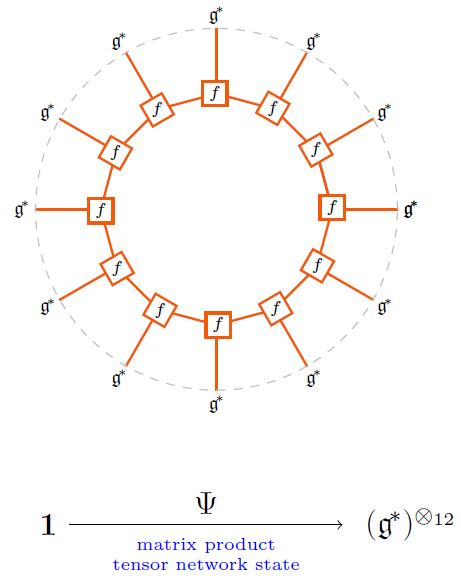# nLab matrix product state

Contents

### Context

#### Monoidal categories

monoidal categories

# Contents

## Idea

What are called matrix product states (MPS) in quantum physics (specifically in solid state physics and in AdS/CFT) are those tensor network states of the form of a ring of tensors all of rank 3.

For example, given a metric Lie algebra $\mathfrak{g}$ (with string diagram-notation as discussed there) its Lie bracket-tensor $f \coloneqq [-,-] \in \mathfrak{g}^\ast \otimes \mathfrak{g}^\ast \otimes \mathfrak{g}$ gives rise to matrix product states of the following form:graphics from Sati-Schreiber 19c

For more see the references at tensor network state.

### General

Review and exposition: